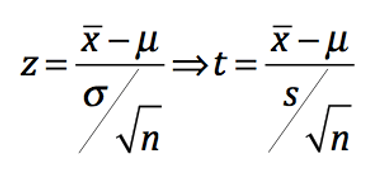## Chapter 7 - Day 7

##### Learning Targets
• Calculate the mean and standard deviation of the sampling distribution of a sample mean and interpret the standard deviation.

• If appropriate, use a Normal distribution to calculate probabilities involving sample means.

##### Activity: How Tall are We?

Be sure to print off or project the Height Data so students can successfully take samples. Also, you will need to prepare two posterboards for dotplots. The first will be the population distribution of heights and the second will be the sampling distribution of sample mean heights. Be sure to use the same scale on both (see scale used in the Activity). This will help to reveal to students that the variability of the sampling distribution is less than the population distribution.​

##### Teaching Tip

Students often forget to divide by the square root of the sample size when finding probabilities involving the sample mean. In the Check Your Understanding problem, make sure to discuss the distinction between #1 and #4 with this in mind. In #1, we are asked to find a probability involving the pregnancy length of one woman (using the population distribution). In #4, we are asked to find a probability involving the mean pregnancy length of a sample of women (using the sampling distribution).

##### Preparing for Inference

In Chapter 9, we will perform a one sample t test for a mean. The standardized test statistic (which will lead us to the P-value) will be given by the following formula. Can you see how students are actually already doing this calculation as a z-score right now? In Chapter 8 we will discover why to use a t distribution instead of a Normal distribution.##### What if the population distribution is not normal?

In today’s lesson, we only dealt with population distributions that were approximately normal. Tomorrow we will consider the case when the population is not Normal.